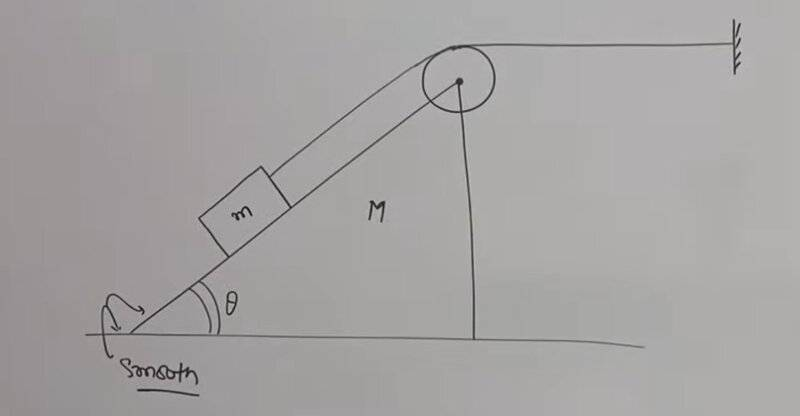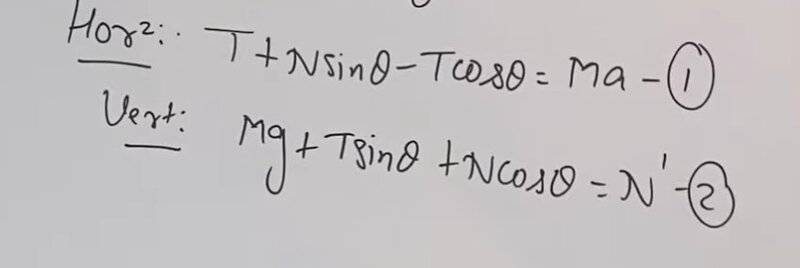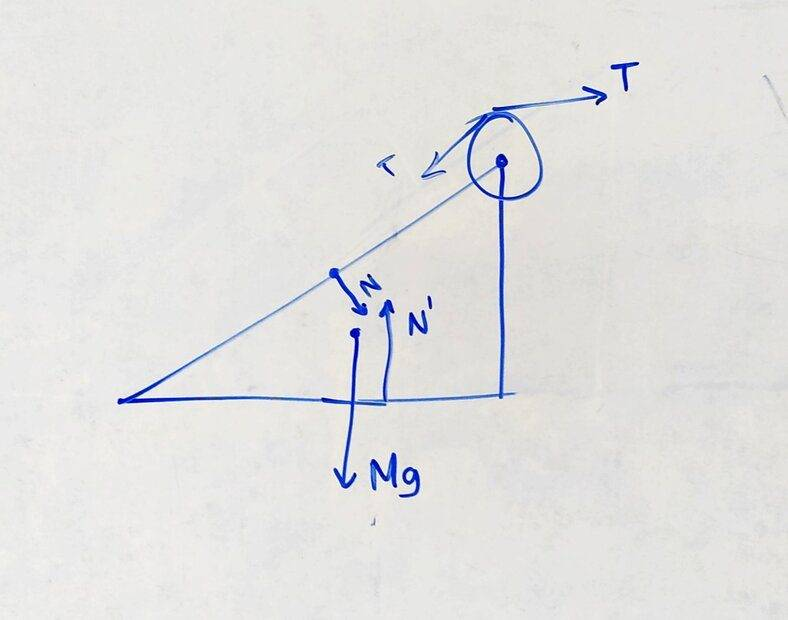# A counterintuitive Laws of Motion problem

• Differentiate it
But if you push the box at an angle relative to the floor, your push will be greater at the corners than in the middle. The reason is that the line of action of the force is not the same at the corners as it is in the middle.

#### Differentiate it

Homework Statement
No friction, massless string, massless pulley and wedge M is not fixed, pulley is attached to M.
Apply Newton's 2nd law on (Mass M + pulley) in Horizontal and Vertical directions.
My question: When I was watching this on YouTube, the teacher summed the forces as usual(I've added a picture). But there is a force of tension acting on the top right of wedge, so wouldn't that create a torque... Or something? What force counters that torque? And why can we just sum the forces as usual rather than thinking about the torques?
Also, I've added an image(blue ink) of how the forces kinda look like on the (Mass M + pulley) system
Relevant Equations
F = maDifferentiate it said:
... But there is a force of tension acting on the top right of wedge, so wouldn't that create a torque... Or something? What force counters that torque? And why can we just sum the forces as usual rather than thinking about the torques?
There are two opposing torques about the bottom right edge of the wedge: one from the weights of the block and the wedge and another from the resultant of the tension at the axis of the pulley.
Simplification of the problem, I guess, assuming the wedge is long enough not to tip over.

•Orodruin
Lnewqban said:
There are two opposing torques about the bottom right edge of the wedge: one from the weights of the block and the wedge and another from the resultant of the tension at the axis of the pulley.
Simplification of the problem, I guess, assuming the wedge is long enough not to tip over.
Even then, is it ok to just sum the forces as is? Also, I don't know if this is a stupid question.. but if a force(like friction) doesn't like, point through the center of mass of an object, why do we sum it as a regular force? And tension here, why are we summing it as a regular force rather than... you know, torque, or something?

Differentiate it said:
Even then, is it ok to just sum the forces as is? Also, I don't know if this is a stupid question.. but if a force(like friction) doesn't like, point through the center of mass of an object, why do we sum it as a regular force? And tension here, why are we summing it as a regular force rather than... you know, torque, or something?
Ok so, is it just for simplicity that they didn't consider any torques?

Differentiate it said:
Even then, is it ok to just sum the forces as is? Also, I don't know if this is a stupid question.. but if a force(like friction) doesn't like, point through the center of mass of an object, why do we sum it as a regular force? And tension here, why are we summing it as a regular force rather than... you know, torque, or something?
Forces sum the same regardless of their application point. Then you generally have torque balance to consider but that is a separate issue. You consider all for es in the force balance and the torques of all forces in the torque balance. You do not add torques with forces.

•Lnewqban
Differentiate it said:
Ok so, is it just for simplicity that they didn't consider any torques?
Probably. What was the original question,?

Last edited:
Orodruin said:
Forces sum the same regardless of their application point. Then you generally have torque balance to consider but that is a separate issue. You consider all for es in the force balance and the torques of all forces in the torque balance. You do not add torques with forces.
I know that we don't add torques with forces, but could you please elaborate on your first sentence? And being realistic, friction also generates a torque which is countered by the normal force. In such a case, we sum the forces as usual. But these forces are causing torques, so how do sum them as regular forces?

Differentiate it said:
Even then, is it ok to just sum the forces as is? Also, I don't know if this is a stupid question.. but if a force(like friction) doesn't like, point through the center of mass of an object, why do we sum it as a regular force? And tension here, why are we summing it as a regular force rather than... you know, torque, or something?
The problem stated no friction.
Forces alone induce linear movement of the center of mass; therefore, you can assume all are applied to the center of mass.
Forces combined with their respective levers induce rotation; therefore, the location of the tail of the vector that represents each force respect to the fulcrum or pivot is important.

Lnewqban said:
There are two opposing torques about the bottom right edge of the wedge: one from the weights of the block and the wedge and another from the resultant of the tension at the axis of the pulley.
You left out the normal force from the ground.
Differentiate it said: